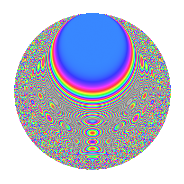# Properties

 Label 5077.2.a.bLevel 5077 Weight 2 Character orbit 5077.a Self dual Yes Analytic conductor 40.540 Analytic rank 1 Dimension 205 CM No

# Related objects

## Newspace parameters

 Level: $$N$$ = $$5077$$ Weight: $$k$$ = $$2$$ Character orbit: $$[\chi]$$ = 5077.a (trivial)

## Newform invariants

 Self dual: Yes Analytic conductor: $$40.5400491062$$ Analytic rank: $$1$$ Dimension: $$205$$ Fricke sign: $$1$$ Sato-Tate group: $\mathrm{SU}(2)$

## $q$-expansion

The dimension is sufficiently large that we do not compute an algebraic $$q$$-expansion, but we have computed the trace expansion.

 $$\operatorname{Tr}(f)(q) =$$ $$205q$$ $$\mathstrut -\mathstrut 25q^{2}$$ $$\mathstrut -\mathstrut 59q^{3}$$ $$\mathstrut +\mathstrut 195q^{4}$$ $$\mathstrut -\mathstrut 44q^{5}$$ $$\mathstrut -\mathstrut 26q^{6}$$ $$\mathstrut -\mathstrut 30q^{7}$$ $$\mathstrut -\mathstrut 75q^{8}$$ $$\mathstrut +\mathstrut 186q^{9}$$ $$\mathstrut +\mathstrut O(q^{10})$$ $$\operatorname{Tr}(f)(q) =$$ $$205q$$ $$\mathstrut -\mathstrut 25q^{2}$$ $$\mathstrut -\mathstrut 59q^{3}$$ $$\mathstrut +\mathstrut 195q^{4}$$ $$\mathstrut -\mathstrut 44q^{5}$$ $$\mathstrut -\mathstrut 26q^{6}$$ $$\mathstrut -\mathstrut 30q^{7}$$ $$\mathstrut -\mathstrut 75q^{8}$$ $$\mathstrut +\mathstrut 186q^{9}$$ $$\mathstrut -\mathstrut 28q^{10}$$ $$\mathstrut -\mathstrut 83q^{11}$$ $$\mathstrut -\mathstrut 108q^{12}$$ $$\mathstrut -\mathstrut 36q^{13}$$ $$\mathstrut -\mathstrut 67q^{14}$$ $$\mathstrut -\mathstrut 63q^{15}$$ $$\mathstrut +\mathstrut 187q^{16}$$ $$\mathstrut -\mathstrut 72q^{17}$$ $$\mathstrut -\mathstrut 57q^{18}$$ $$\mathstrut -\mathstrut 47q^{19}$$ $$\mathstrut -\mathstrut 132q^{20}$$ $$\mathstrut -\mathstrut 35q^{21}$$ $$\mathstrut -\mathstrut 40q^{22}$$ $$\mathstrut -\mathstrut 97q^{23}$$ $$\mathstrut -\mathstrut 49q^{24}$$ $$\mathstrut +\mathstrut 175q^{25}$$ $$\mathstrut -\mathstrut 78q^{26}$$ $$\mathstrut -\mathstrut 227q^{27}$$ $$\mathstrut -\mathstrut 59q^{28}$$ $$\mathstrut -\mathstrut 46q^{29}$$ $$\mathstrut +\mathstrut 30q^{30}$$ $$\mathstrut -\mathstrut 77q^{31}$$ $$\mathstrut -\mathstrut 175q^{32}$$ $$\mathstrut -\mathstrut 74q^{33}$$ $$\mathstrut -\mathstrut 28q^{34}$$ $$\mathstrut -\mathstrut 171q^{35}$$ $$\mathstrut +\mathstrut 171q^{36}$$ $$\mathstrut -\mathstrut 52q^{37}$$ $$\mathstrut -\mathstrut 144q^{38}$$ $$\mathstrut -\mathstrut 54q^{39}$$ $$\mathstrut -\mathstrut 49q^{40}$$ $$\mathstrut -\mathstrut 107q^{41}$$ $$\mathstrut +\mathstrut 7q^{42}$$ $$\mathstrut -\mathstrut 58q^{43}$$ $$\mathstrut -\mathstrut 139q^{44}$$ $$\mathstrut -\mathstrut 89q^{45}$$ $$\mathstrut -\mathstrut 33q^{46}$$ $$\mathstrut -\mathstrut 255q^{47}$$ $$\mathstrut -\mathstrut 202q^{48}$$ $$\mathstrut +\mathstrut 171q^{49}$$ $$\mathstrut -\mathstrut 74q^{50}$$ $$\mathstrut -\mathstrut 63q^{51}$$ $$\mathstrut -\mathstrut 90q^{52}$$ $$\mathstrut -\mathstrut 82q^{53}$$ $$\mathstrut -\mathstrut 51q^{54}$$ $$\mathstrut -\mathstrut 70q^{55}$$ $$\mathstrut -\mathstrut 180q^{56}$$ $$\mathstrut -\mathstrut 70q^{57}$$ $$\mathstrut -\mathstrut 50q^{58}$$ $$\mathstrut -\mathstrut 289q^{59}$$ $$\mathstrut -\mathstrut 105q^{60}$$ $$\mathstrut -\mathstrut 20q^{61}$$ $$\mathstrut -\mathstrut 143q^{62}$$ $$\mathstrut -\mathstrut 119q^{63}$$ $$\mathstrut +\mathstrut 201q^{64}$$ $$\mathstrut -\mathstrut 92q^{65}$$ $$\mathstrut -\mathstrut 3q^{66}$$ $$\mathstrut -\mathstrut 138q^{67}$$ $$\mathstrut -\mathstrut 177q^{68}$$ $$\mathstrut -\mathstrut 67q^{69}$$ $$\mathstrut +\mathstrut 4q^{70}$$ $$\mathstrut -\mathstrut 141q^{71}$$ $$\mathstrut -\mathstrut 138q^{72}$$ $$\mathstrut -\mathstrut 71q^{73}$$ $$\mathstrut -\mathstrut 26q^{74}$$ $$\mathstrut -\mathstrut 251q^{75}$$ $$\mathstrut -\mathstrut 42q^{76}$$ $$\mathstrut -\mathstrut 149q^{77}$$ $$\mathstrut -\mathstrut 6q^{78}$$ $$\mathstrut -\mathstrut 47q^{79}$$ $$\mathstrut -\mathstrut 294q^{80}$$ $$\mathstrut +\mathstrut 193q^{81}$$ $$\mathstrut -\mathstrut 70q^{82}$$ $$\mathstrut -\mathstrut 329q^{83}$$ $$\mathstrut -\mathstrut 40q^{84}$$ $$\mathstrut -\mathstrut 45q^{85}$$ $$\mathstrut -\mathstrut 83q^{86}$$ $$\mathstrut -\mathstrut 139q^{87}$$ $$\mathstrut -\mathstrut 45q^{88}$$ $$\mathstrut -\mathstrut 163q^{89}$$ $$\mathstrut -\mathstrut 116q^{90}$$ $$\mathstrut -\mathstrut 141q^{91}$$ $$\mathstrut -\mathstrut 204q^{92}$$ $$\mathstrut -\mathstrut 91q^{93}$$ $$\mathstrut -\mathstrut 8q^{94}$$ $$\mathstrut -\mathstrut 173q^{95}$$ $$\mathstrut -\mathstrut 53q^{96}$$ $$\mathstrut -\mathstrut 147q^{97}$$ $$\mathstrut -\mathstrut 156q^{98}$$ $$\mathstrut -\mathstrut 157q^{99}$$ $$\mathstrut +\mathstrut O(q^{100})$$

## Embeddings

For each embedding $$\iota_m$$ of the coefficient field, the values $$\iota_m(a_n)$$ are shown below.

For more information on an embedded modular form you can click on its label.

Label $$a_{2}$$ $$a_{3}$$ $$a_{4}$$ $$a_{5}$$ $$a_{6}$$ $$a_{7}$$ $$a_{8}$$ $$a_{9}$$ $$a_{10}$$
1.1 −2.81633 −3.09812 5.93172 −0.550838 8.72533 −3.77340 −11.0730 6.59836 1.55134
1.2 −2.81174 −2.29296 5.90586 2.02073 6.44719 2.09923 −10.9822 2.25765 −5.68176
1.3 −2.78060 0.0708990 5.73173 −3.99378 −0.197142 2.14019 −10.3764 −2.99497 11.1051
1.4 −2.77684 2.42901 5.71081 0.850994 −6.74496 0.0263654 −10.3043 2.90008 −2.36307
1.5 −2.77001 −0.453815 5.67294 −2.74837 1.25707 −3.66610 −10.1741 −2.79405 7.61300
1.6 −2.75099 0.881000 5.56794 −0.176984 −2.42362 4.01245 −9.81537 −2.22384 0.486880
1.7 −2.72893 1.44573 5.44706 1.77131 −3.94530 −0.914980 −9.40679 −0.909861 −4.83377
1.8 −2.70901 2.53540 5.33875 −3.58245 −6.86844 1.35106 −9.04473 3.42827 9.70491
1.9 −2.65566 −0.00621299 5.05254 3.61372 0.0164996 −2.37510 −8.10651 −2.99996 −9.59681
1.10 −2.63506 −2.68532 4.94352 −3.56465 7.07597 1.99537 −7.75633 4.21094 9.39304
1.11 −2.63322 −3.42055 4.93387 2.45426 9.00708 4.09681 −7.72553 8.70017 −6.46261
1.12 −2.62320 −1.94038 4.88119 −2.34759 5.09001 2.45052 −7.55796 0.765079 6.15820
1.13 −2.61107 −0.462954 4.81767 −1.81913 1.20880 2.59865 −7.35711 −2.78567 4.74986
1.14 −2.58891 3.03601 4.70247 −2.12687 −7.85998 3.03640 −6.99646 6.21738 5.50629
1.15 −2.58762 0.766219 4.69576 1.84822 −1.98268 −5.04954 −6.97561 −2.41291 −4.78248
1.16 −2.54248 2.75174 4.46418 −3.07759 −6.99622 −3.90745 −6.26513 4.57206 7.82470
1.17 −2.48215 −2.18420 4.16105 −1.67259 5.42150 2.74743 −5.36403 1.77073 4.15162
1.18 −2.47319 −1.27283 4.11668 3.46270 3.14796 5.16648 −5.23495 −1.37990 −8.56393
1.19 −2.46210 −1.32036 4.06192 0.169195 3.25085 −3.54943 −5.07665 −1.25666 −0.416575
1.20 −2.46112 −3.25745 4.05713 −3.21291 8.01698 −0.720862 −5.06284 7.61098 7.90737
See next 80 embeddings (of 205 total)
 $$n$$: e.g. 2-40 or 990-1000 Embeddings: e.g. 1-3 or 1.205 Significant digits: Format: Complex embeddings Normalized embeddings Satake parameters Satake angles

## Inner twists

This newform does not have CM; other inner twists have not been computed.

## Atkin-Lehner signs

$$p$$ Sign
$$5077$$ $$1$$

## Hecke kernels

This newform can be constructed as the kernel of the linear operator $$T_{2}^{205} + \cdots$$ acting on $$S_{2}^{\mathrm{new}}(\Gamma_0(5077))$$.